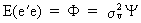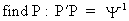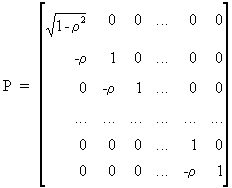##### Linear Regression# Online Econometrics Textbook - Regression Extensions - Assumption Violations of Linear Regression - Autocorrelation in Linear Regression

#### III.I.2 Autocorrelation in Linear Regression

How can we solve the problem of autocorrelation as in (III.I.2-1))?(III.I.2-1)

We can write (III.I.2-1) in terms of variances(III.I.2-2)

but since we may assume homoskedasticity it follows that(III.I.2-3)

Using (III.I.2-1) the covariance with a lag of 1 period is(III.I.2-4)

In general the covariance with lag k can be written as(III.I.2-5)

and the covariance matrix of the residuals as(III.I.2-6)

which therefore yields(III.I.2-7)

The GLS solution to this problem, if r is assumed to be known, is a tree stage procedure

stage 1(III.I.2-8)

stage 2(III.I.2-9)

stage 3(III.I.2-10)

using equation (III.I-8).

Remark that(III.I.2-11)

and that(III.I.2-12)

which implies(III.I.2-13)There are two other alternatives to solve the problem of (III.I.2-1): lagged variables, and distributed lags.

Sometimes lagged endogenous and/or exogenous variables can be introduced in order to reduce the autocorrelation problem. This is a quite flexible tool with however, one important drawback. The introduction of each additional variable reduces the degrees of freedom, whereas the use of a lagged variable mostly forces the researcher to reduce the sample range which also implies a loss of degrees of freedom (º T - K). Also remember that OLS is not an efficient estimator in the context of autocorrelation. Even worse is the estimation of parameters of lagged endogenous variables in the context of autocorrelation induced by unobserved variables (or wrong specification): in this case the OLS estimates will be biased.

Distributed lags can also be used to solve problem of (III.I.2-1). A huge advantage over the lagged variables method is that the distributed lags do not incur as large a loss of degrees of freedom as the first method. A drawback of this method could be the fact that the estimation procedure is not always straightforward and sometimes even difficult. The method of distributed lags will be discussed in detail in later sections.

In econometrics the Durbin-Watson statistic (c.q. d statistic) is frequently used(III.I.2-14)

Since(III.I.2-15)

eq. (III.I.2-14) can be rewritten as(III.I.2-16)

for large samples, where r is the correlation between the residual at period t and the residual at t-1. Note that d = 2 in case there is no (first order) autocorrelation in the residual series.

The Durbin-Watson statistic will fail when time series data are strongly seasonal. In this case an adapted d statistic, for a seasonal period s, can be defined as(III.I.2-17)

which is in fact an autocorrelation coefficient of order s.

The d statistic will, in general, also fail in case a regression equation under OLS is estimated with the lagged endogenous variable variable used as regressor. In this case Durbin suggests alternatives (the so-called h statistics) such as(III.I.2-18)Another way to detect AR(p) autocorrelation is the Breusch-Godfrey test based on a test-equation: the residuals are explained by its lagged values (p is the number of lags included in the test-equation) and all exogenous variables of the original equation. The presence of AR(p) autocorrelation is tested by the use of a Chi-square-statistic (c.q. (T-p)*R-square).

Below you ll find an example of how the Breusch-Godfrey test can be applied to test for AR(p) autocorrelation (this test is applied to our example-equation):

```Breusch-Godfrey test for AR(p) residuals by Least Squares:

Estimation with OLS:

Endogenous variable =  Residuals

Variable                     Parameter                S.E.      T-STAT
employ                   -6.574423604           7.235339332     -0.909
expend                   +2.286765988           2.796278607     +0.818
const                   +82.24312207          278.872104        +0.295
resid(-1)                -0.449639967           0.2411178087    -1.86
resid(-2)                -0.2887649456          0.194556882     -1.48
resid(-3)                -5.403469274e-002      0.2080984616    -0.26

2-tail-t at 95 percent = 2.042
1-tail-t at 95 percent = 1.697
R-squared of stationary series = 0.1647233405
Durbin-Watson                  = 1.999045701
Degrees of freedom             = 30
Variance of regression         = 1052262.317
Standard Error of regression   = 1025.79838
Sum of Squared Residuals       = 31567869.52

Correlation matrix of parameters:

+1.00  -0.91  -0.51  +0.67  -0.08  +0.34
-0.91  +1.00  +0.21  -0.61  +0.14  -0.27
-0.51  +0.21  +1.00  -0.31  -0.00  -0.21
+0.67  -0.61  -0.31  +1.00  +0.16  +0.42
-0.08  +0.14  -0.00  +0.16  +1.00  +0.20
+0.34  -0.27  -0.21  +0.42  +0.20  +1.00

(T-p)*R-square =  5.435870235
Critical Chi-square value (95%) = 7.81 (degrees of freedom = 3)

```

Many other tests are available to diagnose autocorrelation problems, many of which belong to the domain of time series analysis (chapter 5).No news at the moment...© 2000-2018 All rights reserved. All Photographs (jpg files) are the property of Corel Corporation, Microsoft and their licensors. We acquired a non-transferable license to use these pictures in this website.
The free use of the scientific content in this website is granted for non commercial use only. In any case, the source (url) should always be clearly displayed. Under no circumstances are you allowed to reproduce, copy or redistribute the design, layout, or any content of this website (for commercial use) including any materials contained herein without the express written permission.

Information provided on this web site is provided "AS IS" without warranty of any kind, either express or implied, including, without limitation, warranties of merchantability, fitness for a particular purpose, and noninfringement. We use reasonable efforts to include accurate and timely information and periodically updates the information without notice. However, we make no warranties or representations as to the accuracy or completeness of such information, and it assumes no liability or responsibility for errors or omissions in the content of this web site. Your use of this web site is AT YOUR OWN RISK. Under no circumstances and under no legal theory shall we be liable to you or any other person for any direct, indirect, special, incidental, exemplary, or consequential damages arising from your access to, or use of, this web site.

Contributions and Scientific Research: Prof. Dr. E. Borghers, Prof. Dr. P. Wessa
Please, cite this website when used in publications: Xycoon (or Authors), Statistics - Econometrics - Forecasting (Title), Office for Research Development and Education (Publisher), http://www.xycoon.com/ (URL), (access or printout date).